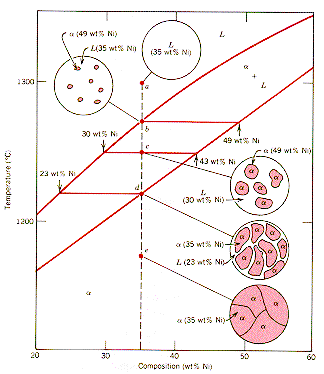Phase Diagrams The Lever Rule · The microstructure and compositions of the phases present are shown on the partial diagram for a Cu-35 wt% Ni alloy ( 65 wt% Cu, 35 wt% Ni) · The equilibrium mass fraction of each phase present in a two phase zone is estimated using the "Lever Rule." · For a zone with two phases A, B present, total mass conservation requires: WA + WB = 1 where WI is the mass fraction of I. · From conservation of constituents:  WA CA + WB CB = C0 1From: Callister, "Materials Science and Engineering," Wiley (1997)